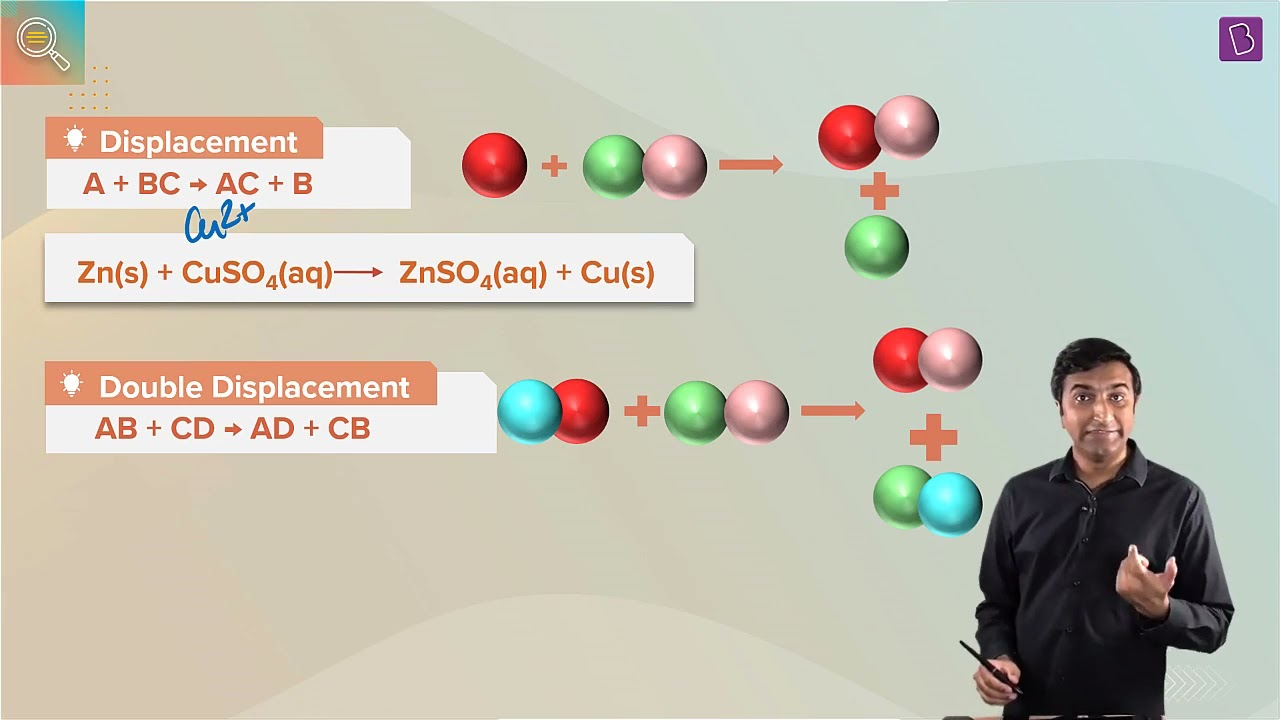# What is the difference between single displacement reaction double displacement reaction?The reaction in which an atom or a set of atoms are displaced by another atom in a molecule is called displacement reaction. For instance, when Iron is added to a copper sulfate solution, it displaces the copper metal.

A + B – C → A – C + B

The above equation exists when A is more reactive than B.

The reaction in which two atoms or a group of atoms are displaced by another atom in a molecule is called a double displacement reaction.

A-B + C-D → A-C + B-D

A and B have to be either:

• Halogens where C indicates a cation.
• Different metals wherein C indicates an anion.

The difference between displacement reaction double displacement reaction is tabulated below:

 Single Displacement reaction Double displacement reaction In a displacement reaction, a more reactive element replaces a less reactive element from a compound. In a double displacement reaction, two atoms or a group of atoms switch places to form new compounds. In a displacement reaction, one displacement takes place. A + BX → AX + B In a double displacement reaction, two displacements take place to complete the reaction. AB + CD → AC + BD Change in color takes place with no precipitate forms. Precipitate is formed. Metals react with the salt solution of another metal. Salt solutions of two different metals react with each other. Examples: 2KI + Cl2 → 2KCl + I2 is a displacement reaction. CuSO4 + Zn → ZnSO4 + Cu is also a displacement reaction. Examples:  Na2SO4 + BaCl2 → BaSO4 + 2NaCl is a double displacement reaction. 2KBr + BaCl2 → 2KCl + BaBr2 is also a double displacement reaction.

4.5 (185)(383)(56)

#### Choose An Option That Best Describes Your Problem

Thank you. Your Feedback will Help us Serve you better.

1. It was very helpful. Thanks

• Kanupriya

Very very use fullllll

• Ankita

• Srishti

Byjus help me to clear my all concept and it’s help me to learn difficult thing s with easier way thank you byjus to be my learner🙂

2. This was the perfect answer for me
This helped me a lot
Thank you so much for such a nice answer☺️☺️

• Harshit tyagi

Very usefull

• Anjali jha

5. Very easy for understanding this answer

6. Akshit gujjar

So good

7. Dhipti Sharma

8. Gaurav Goyal

Very nice and useful answer which helped me in understanding this topic more clearly.

Thank you to BYJU’S.

9. Sujhat ali

10. Subramoniam C B

Thank, team byjus for giving this answer in simple and conceptual way.

12. Gunjan sehrawat

It is a perfect answer of this question , also an simple , conceptual and it clears all the things very well. It gives a proper way to express each and every things about these reactions .
So thank you byjus for giving this answer.

13. Sushree

It is so much useful one

15. Imran bengeri

Useful for me

16. It is very usefull

17. Good answer which is very useful

18. shizakashif

Thanks for helping me

19. Dakssh Dixit

21. Pramugdha Gupta

22. Thanks for this information information

23. Anjali jha

Very